# Question 21 3 pts If you were interesting in determining a company's ability to pay its...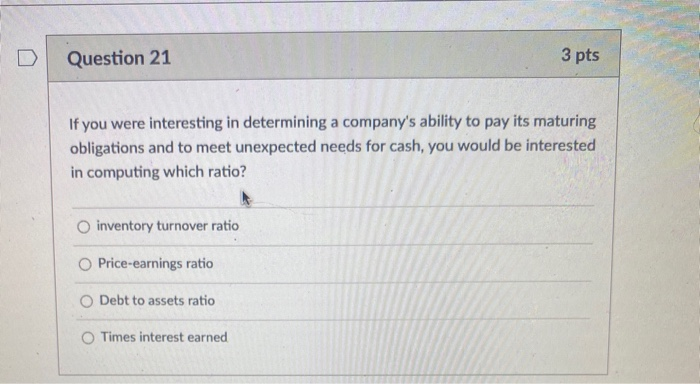Question 21 3 pts If you were interesting in determining a company's ability to pay its maturing obligations and to meet unexpected needs for cash, you would be interested in computing which ratio? inventory turnover ratio Price-earnings ratio Debt to assets ratio Times interest earned

This Homework Help Question: "Question 21 3 pts If you were interesting in determining a company's ability to pay its..." No answers yet.

We need 10 more requests to produce the answer to this homework help question. Share with your friends to get the answer faster!

0 /10 have requested the answer to this homework help question.

Once 10 people have made a request, the answer to this question will be available in 1-2 days.
All students who have requested the answer will be notified once they are available.
##### Add Answer to: Question 21 3 pts If you were interesting in determining a company's ability to pay its...
Similar Homework Help Questions
• ### Which of the following measures a company's ability to pay its current liabilities? earnings per share...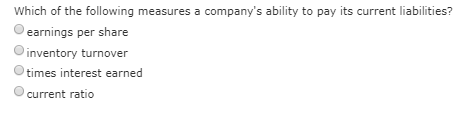Which of the following measures a company's ability to pay its current liabilities? earnings per share inventory turnover times interest earned current ratio

• ### Clear All Current ratio A measure of a company's ability to pay its short-term liabilities out...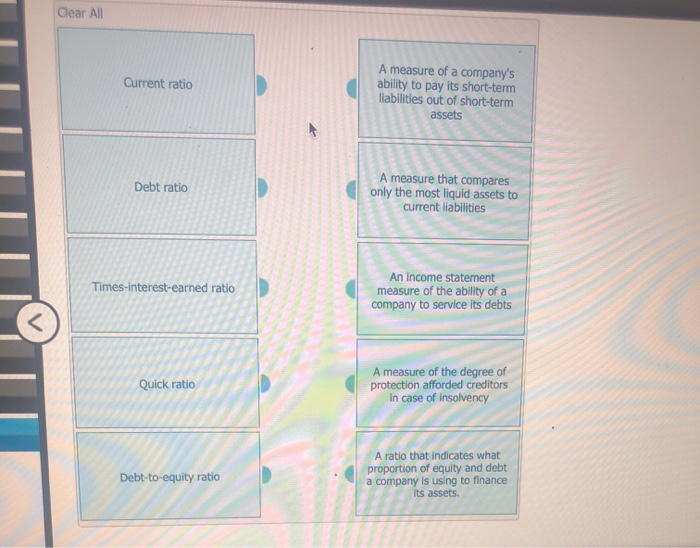Clear All Current ratio A measure of a company's ability to pay its short-term liabilities out of short-term assets Debt ratio A measure that compares only the most liquid assets to current liabilities Times-interest-earned ratio An income statement measure of the ability of a company to service its debts Quick ratio A measure of the degree of protection afforded creditors in case of insolvency Debt-to-equity ratio A ratio that indicates what proportion of equity and debt a company is using...

• ### Question Help A company's ability to pay liabilities with current assets is measured by which of...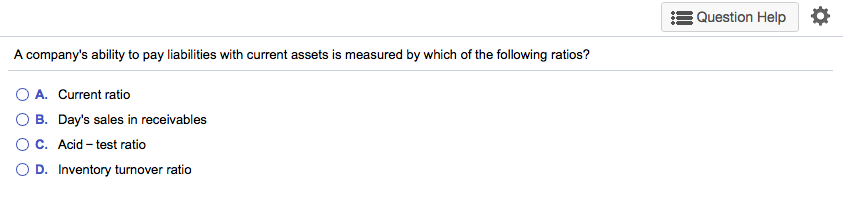Question Help A company's ability to pay liabilities with current assets is measured by which of the following ratios? O A. Current ratio O B. Day's sales in receivables O C. Acid - test ratio OD. Inventory turnover ratio

• ### Match each ratlo that follows to its use items a-n). Items may be used more than...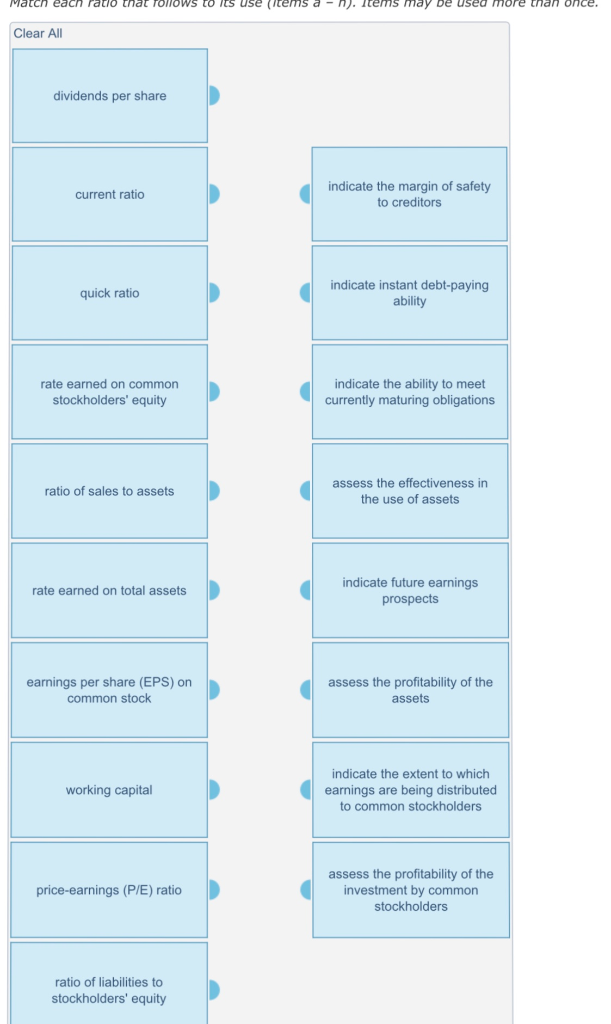Match each ratlo that follows to its use items a-n). Items may be used more than once. Clear All dividends per share current ratio indicate the margin of safety to creditors quick ratio indicate instant debt-paying ability rate earned on common stockholders' equity indicate the ability to meet currently maturing obligations ratio of sales to assets assess the effectiveness in the use of assets rate earned on total assets indicate future earnings prospects earnings per share (EPS) on common stock...

• ### 1 pts In performing a vertical analysis of an income statement, which of the following is...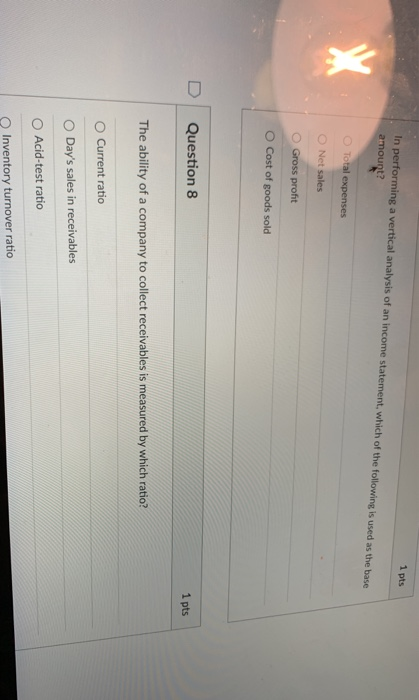1 pts In performing a vertical analysis of an income statement, which of the following is used as the base amount? X Total expenses Net sales Gross profit O Cost of goods sold Question 8 1 pts The ability of a company to collect receivables is measured by which ratio? Current ratio Day's sales in receivables Acid-test ratio Inventory turnover ratio Question 9 Working capital is a measure of: A company's ability to pay its current obligations The amount of...

• ### The Current Ratio assesses a company's ability to pay its debts in the near term and...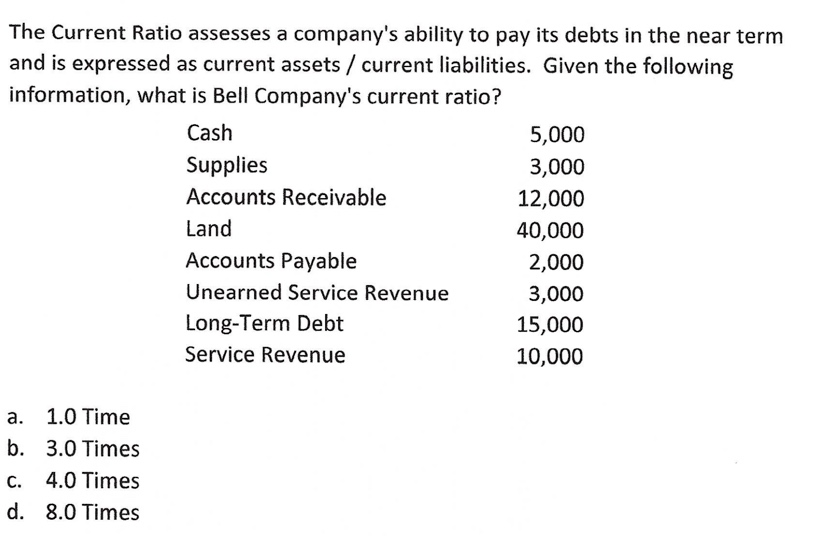The Current Ratio assesses a company's ability to pay its debts in the near term and is expressed as current assets / current liabilities. Given the following information, what is Bell Company's current ratio? Cash 5,000 Supplies 3,000 Accounts Receivable 12,000 Land 40,000 Accounts Payable 2,000 Unearned Service Revenue 3,000 Long-Term Debt 15,000 Service Revenue 10,000 a. 1.0 Time b. 3.0 Times C. 4.0 Times d. 8.0 Times

• ### Houle Furniture Company has asked you to determine whether the company's ability to pay its current...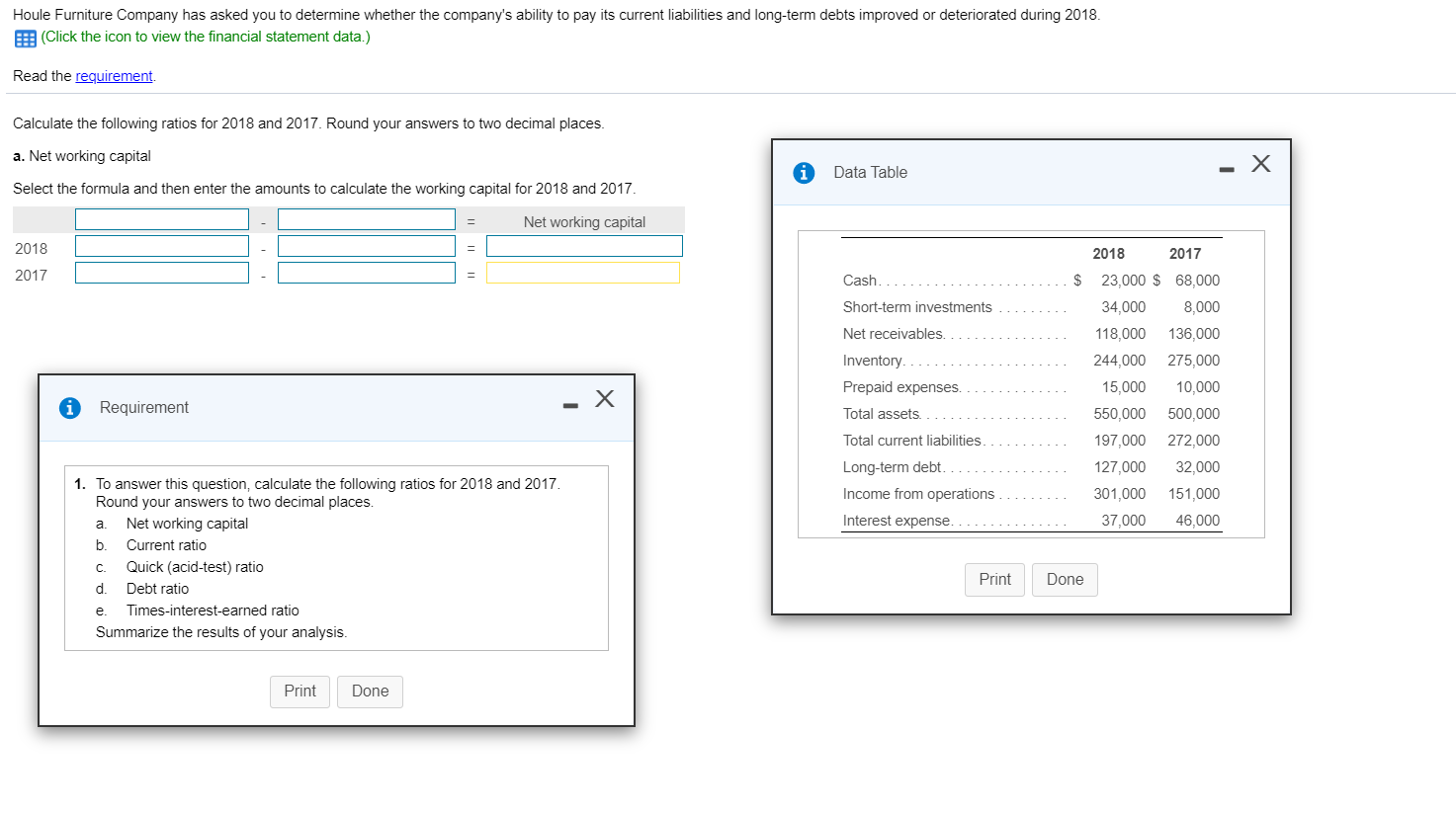Houle Furniture Company has asked you to determine whether the company's ability to pay its current liabilities and long-term debts improved or deteriorated during 2018. (Click the icon to view the financial statement data.) Read the requirement. Calculate the following ratios for 2018 and 2017. Round your answers to two decimal places. a. Net working capital A Data Table Select the formula and then enter the amounts to calculate the working capital for 2018 and 2017. Net working capital 2018...

• ### Autumn Frames has asked you to determine whether the company's ability to pay current liabilities and...Autumn Frames has asked you to determine whether the company's ability to pay current liabilities and total liabilities improved or deteriorated during 2017 To answer that question, compute these ratios for 2017 and 2016, using the following data (Click the icon to view the financial information) Read the requirements a. Current ratio Enter the formula on the first line, then calculate the ratio for each year (Round your answers to two decimal places) Current rato 2017 2010 - X Data...

• ### Multiple choice help

1) Which of the following ratios should be used in evaluating the effectiveness with which the company uses its assets?Receivables TurnoverPayout Ratioa.YesYesb.NoNoc.YesNod.NoYesa) cb) ac) bd) d2)Which of the following ratios is(are) useful in assessing a company's ability to meet current maturing orshort-term obligations?Acid-Test RatioDebt to Total Assets Ratioa.NoNob.NoYesc.YesYesd.YesNoa) bb) dc) ad) c3)How is the average inventory used in the calculation of each of the following?Acid-Test (Quick) RatioInventory Turnover Ratioa.NumeratorNumeratorb.NumeratorDenominatorc.Not UsedDenominatord.Not UsedNumeratora) cb) dc) ad) bThank you for your help

• ### Q1. Hill Roy Corporation has asked the accounts department to determine whether the company's ability to...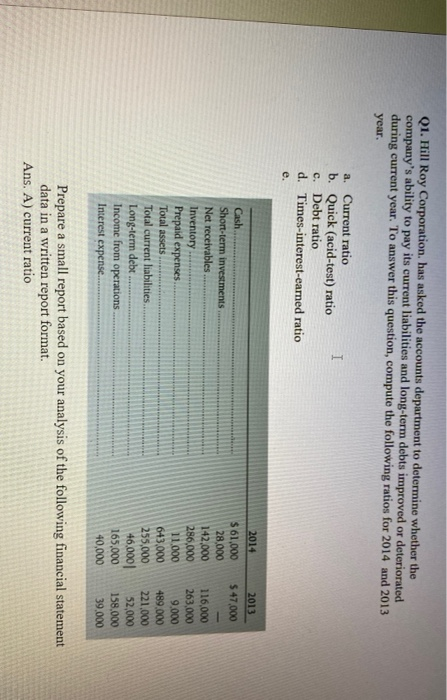Q1. Hill Roy Corporation has asked the accounts department to determine whether the company's ability to pay its current liabilities and long-term debts improved or deteriorated during current year. To answer this question, compute the following ratios for 2014 and 2013 year. I a. Current ratio b. Quick (acid-test) ratio c. Debt ratio d. Times-interest-earned ratio e. 2013 \$ 47,000 Cash Short-term investments Net receivables Inventory Prepaid expenses Total assets Total current liabilities Long-term debt. Income from operations Interest expense....

Free Homework App